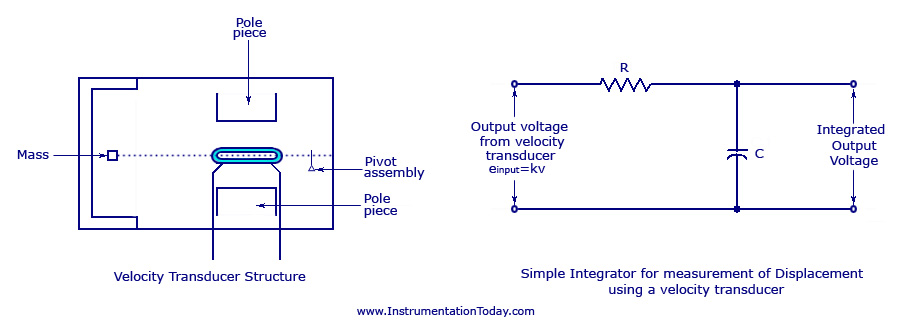UXDE dot Net Wordpress Themes

# Velocity Transducer

#### v=N(d∅/dt) N – Number of turns of the coil d∅/dt – Rate of change of flux in the coil

The voltage produced will be proportional to any type of velocities like linear, sinusoidal or random.

#### The damping is obtained electrically. Thus, we can assume a very high stability under temperature conditions. The basic arrangement of a velocity sensor is shown below.

Velocity Transducer Arrangement

The figure shows a moving coil kept under the influence of two pole pieces. The output voltage is taken across the moving coil. The moving coil is kept balanced for a linear motion with the help of a pivot assembly.Velocity Transducer

Measurement of Displacement Using Velocity Transducer
We know that velocity is the derivative of displacement with respect to time. Similarly, displacement is the time integral of velocity. Thus, a velocity transducer can be used to find the displacement of an object. All we have to do is add an integrating circuit to the velocity transducer arrangement. This is shown in the figure above.
You may also like: Acceleration Transducer
The output voltage (einput) of the transducer can be represented as the product of a constant k and the instantaneous velocity v. If the velocity varies sinusoidally according to its frequency f, and has a peak value V, then the output voltage can be written as

einput = kV2πft
Capacitor Reactance Xc = 1/2πfc

When the value of frequency f is too low, the value of Xc will be very large. So, the integrated output voltage, eoutput will be proportional to einput and so will also be proportional to the velocity v. When the value of frequency becomes high, the value of Xc will become small. Thus, the integrated output voltage can be written as

eoutput = einput/JwCR
eoutput = KV/wCR Sin(wt-90°)

This shows that the value of integrator output lags behind the value of the input voltage by 90 degrees. For a given value of velocity amplitude V, the integrator output is inversely proportional to frequency w.